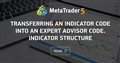# Needing help on locating peak and trough prices and finding the corresponding RSI values, much appreciated!233

Hello, I am trying to design an EA to trade on divergence between the prices and RSI values. I have read this article https://www.mql5.com/en/articles/1456 and borrowed some logic in my coding to transfer an indicator code into an EA code. However, I have two questions and I would greatly appreciate help on them!

1. I have set up "Indicator Buffers Emulation" and int "limit, MaxBar, i, counted_bars", but I am not sure if I am coding it correctly. I would like peak[i] to represent a peak price and peak[i+1] to represent the peak price prior to peak[i], the same for trough prices (Should I use a different int here from "i"?). Could anyone take a look at my code and see if the arrays and the ints are correctly set up for my EA?

2. I would like to find RSI values corresponding to the peak/trough prices, for example, RSIpeak[i] and RSIpeak[i+1], but I don't know how I should write this scheme, I have set up an Array as Series for RSI then I don't know what to do next, as it is not certain how many bars passed between peak[i] and peak[i+1]?

Thanks again for your time reading my question and my deep gratitude for anyone who can help me solve these two problems.

```//---- buffers
double peak[];
double trough[];
double rsi[];```

```int start()
{
// GETTING THE AMOUNT OF ALL BARS OF THE CHART
int IBARS = iBars(NULL, 0);

// INDICATOR BUFFERS EMULATION
if(ArraySize(peak) < IBARS)
{
ArraySetAsSeries(peak, false);
ArraySetAsSeries(trough, false);
ArraySetAsSeries(rsi, false);
//----
ArrayResize(peak, IBARS);
ArrayResize(trough, IBARS);
ArrayResize(rsi, IBARS);
//----
ArraySetAsSeries(peak, false);
ArraySetAsSeries(trough, false);
ArraySetAsSeries(rsi, false);
}
// INSERTION OF A STATIC INTEGER MEMORY VARIABLE
static int IndCounted;

//----+ Insertion of integer variables and GETTING ALREADY CALCULATED BARS
int limit, MaxBar, i, counted_bars = IndCounted;
//----+ REMEMBERING THE AMOUNT OF ALL BARS OF THE CHART
IndCounted = IBARS - 1;
//---- defining the number of the oldest bar,
//     starting from which new bars will be recalculated
limit = IBARS - counted_bars - 1;
//---- defining the number of the oldest bar,
//     starting from which new bars will be recalculated
MaxBar = IBARS - 1;
//---- initialization of zero
if(limit > MaxBar)
{
limit = MaxBar;
for(i = IBARS - 1; i >= 0; i--)
{
peak[i] = 0.0;
trough[i] = 0.0;
rsi[i] = 0.0;
}
}
//----+ THE FIRST CYCLE OF INDICATOR CALCULATION
for(i = IBARS - counted_bars; i >= 0; i--)
{
// Finding the peak prices
if(iHighest(NULL,0,MODE_HIGH,5,1)==2)
peak[i]=High[iHighest(NULL,0,MODE_HIGH,5,1)];
}
//----+ THE SECOND CYCLE OF INDICATOR CALCULATION
for(i = IBARS - counted_bars; i >= 0; i--)
{
// Finding the trough prices
if(iLowest(NULL,0,MODE_HIGH,5,1)==2)
trough[i]=Low[iLowest(NULL,0,MODE_HIGH,5,1)];
}```Transferring an Indicator Code into an Expert Advisor Code. Indicator Structure
• www.mql5.com
I will repeat, that the above method is for EAs working only on closed bars, i.e. on all bars except the zero one! If you are seriously going to use your EA in real trading and trust it your money, you should carefully check all the details in your Expert Advisor, as well as the indicators with which the EA works. Moreover you should do it...